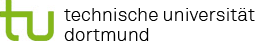You are here:

### Project P5: Experimental design for exposure time-dose curves

In project P5, optimal designs will be derived for the models developed in projects P2 and P3, respectively.

It is well known that optimal design of experiments can substantially improve the statistical inference in regression analysis (Dette and Schorning, 2016). In the context of toxicology, most of the literature concentrates on dose-response curves, where the focus lies on the determination of different doses and corresponding sample size allocations so that the curve under consideration and further resulting parameters of interest, such as the maximum tolerated dose, can be estimated precisely (Dette et al., 2010, among many others).

There are several approaches to determine optimal designs for time dependent data (e.g., Schorning et al., 2020). However, in contrast to the dose-response setting, in which the data are usually assumed to be independent, one has to deal with correlated data in the context of timedependent data. Consequently, the theory developed for independent data cannot be applied in this case.

However, optimal designs for exposure time-dose responses or dose-time response models that both provide the optimal doses and the optimal time points (or slots) have not been derived so far. One approach in dealing with optimal designs for dose-time response models has been introduced by Lange and Schmidli (2014), who derived optimal designs for the doses and the sample size allocations under the assumption that the time component in these models can be influenced by the experimenter. In contrast, Fang and Hedayat (2008) and Dette et al. (2012) concentrate on determining optimal time points in such models, whereas doses are fixed.

The goal of this project is to develop new methods that can be used to derive the best doses and time points (or slots) connected to the best allocation of sample sizes, resulting in the most precise estimation of dose-time response models or exposure time-dose curves, respectively.

In a first step, we will investigate different strategies to derive optimal designs for time-dose response models, where we will use the aforementioned respective results for independent data. Starting with the assumption that both the dose and the time component are independent we will consider new optimality criteria for this special kind of models. On the one hand, we will define criteria inspired by classical optimality criteria (like the D- or A- optimality). On the other hand, we will develop criteria, whose optimisation results in a most precise estimation of parameters of interest, e.g. of the ALEC (absolute lowest effective concentration) connected to a fixed exposure time or of the absolute maximum exposure time connected to a fixed dose. Moreover, we will compare our results to sequential procedures in which we first fix either the dose or the time component. In a second step, we will assume that data are correlated with respect to the time component, and we will generalize our results to this setting using the approaches developed by Schorning et al. (2020). In this context, we will also compare the optimal designs under the assumption of independent and dependent time data.

Finally, we will extend these results to the models developed in the projects P2 and P3. Since these models will be complex, the optimal designs according to the developed criteria will have to be calculated numerically. Moreover, we will use the models developed in projects P2 and P3 to analyse the performance of the numerically calculated designs: More precisely, we will first use a simulation study, in which we compare their performance to designs classically used in toxicology. In a second step, the results will be evaluated on real data provided by IfADo. Experiments with a large number of doses, exposure times, and replicates will be performed. The true model can then be precisely estimated on the full data set, and the performance of the designs can be compared on respective subsets of the full data set. The newly developed designs can be used for the models developed in project P2 and P3.

#### References

• Dette H, Kiss C, Bevanda M, Bretz F (2010). Optimal designs for emax, log-linear and exponential models. Biometrika 97 (2), 513-518, doi: 10.1093/biomet/asq020.
• Dette H, Pepelyshev A, Wong WK (2012). Optimal designs for composed models in pharmacokinetic-pharmacodynamic experiments. J Pharmacokinetics and Pharmacodynamics 39 (3), 295-311, doi: 10.1007/s10928-012-9251-7.
• Dette H, Schorning K (2016). Optimal designs for comparing curves. The Annals of Statistics 44(3), 1103-1130, doi: 10.1214/15-AOS1399.
• Fang X, Hedayat AS (2008). Locally D-optimal designs based on a class of composed models resulted from blending emax and one-compartment models. The Annals of Statistics 36 (1), 428-444, doi: 10.1214/009053607000000776.
• Lange MR, Schmidli H (2014). Optimal design of clinical trials with biologics using dose-timeresponse models. Statistics in Medicine 33(30), 5249-5264, doi: 10.1002/sim.6299. Schorning K, Dette H, Konstantinou M (2020). Optimal designs for series estimation in nonparametric regression with correlated data. To appear in: Statistica Sinica.